Скачать презентацию Completeness and Complexity of Bounded Model Checking Ed

1c05f511c3bd10d025d4e5bb894a6ccc.ppt

• Количество слайдов: 27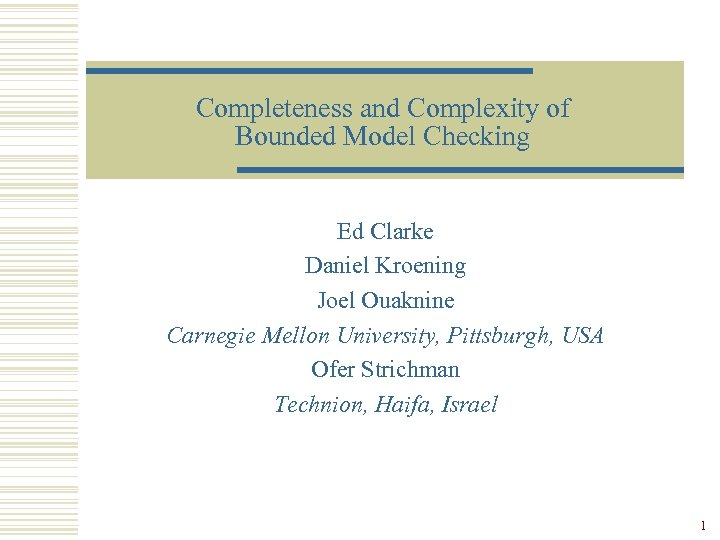Completeness and Complexity of Bounded Model Checking Ed Clarke Daniel Kroening Joel Ouaknine Carnegie Mellon University, Pittsburgh, USA Ofer Strichman Technion, Haifa, Israel 1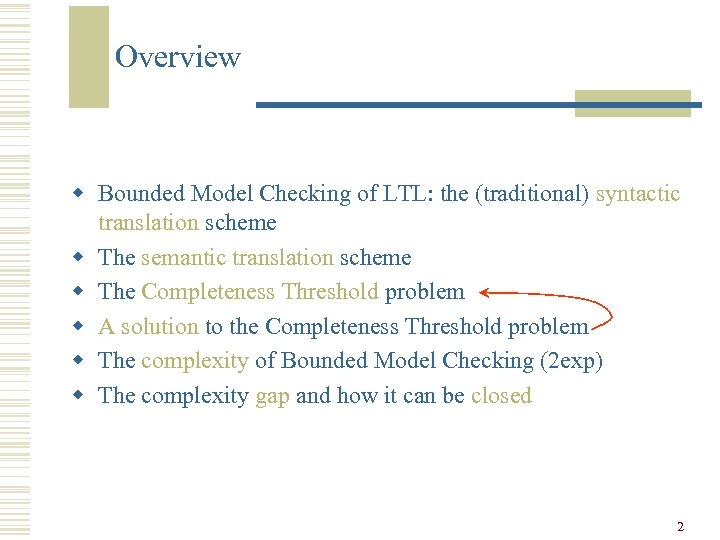Overview w Bounded Model Checking of LTL: the (traditional) syntactic translation scheme w The semantic translation scheme w The Completeness Threshold problem w A solution to the Completeness Threshold problem w The complexity of Bounded Model Checking (2 exp) w The complexity gap and how it can be closed 2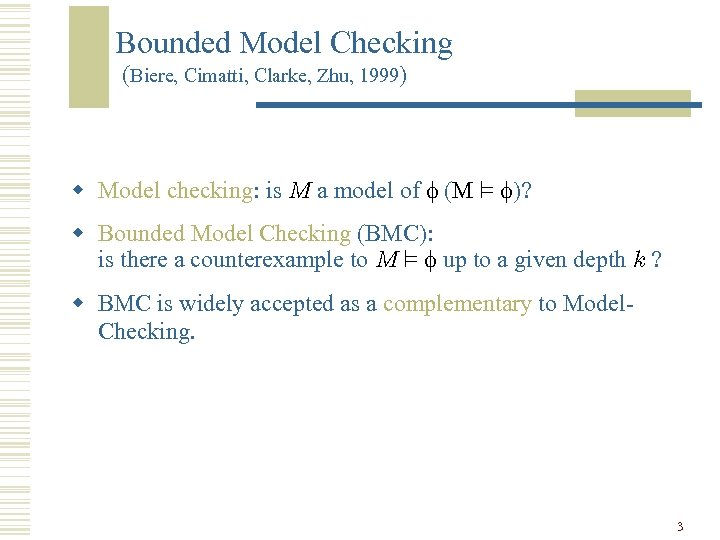Bounded Model Checking (Biere, Cimatti, Clarke, Zhu, 1999) w Model checking: is M a model of (M ² )? w Bounded Model Checking (BMC): is there a counterexample to M ² up to a given depth k ? w BMC is widely accepted as a complementary to Model. Checking. 3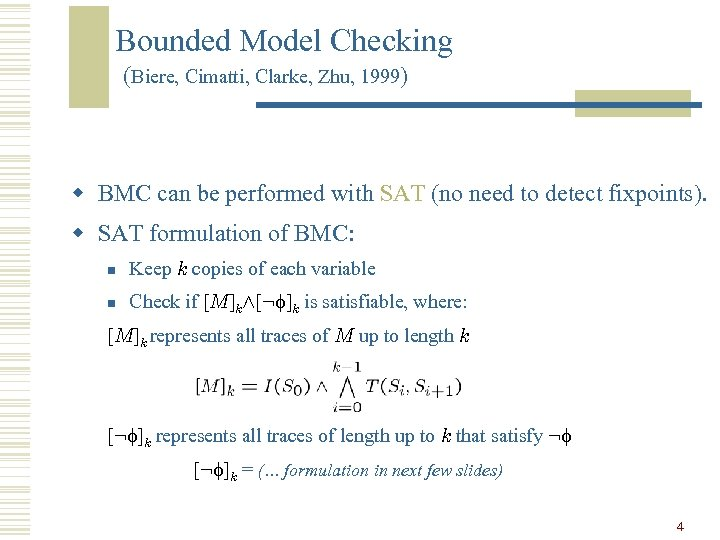Bounded Model Checking (Biere, Cimatti, Clarke, Zhu, 1999) w BMC can be performed with SAT (no need to detect fixpoints). w SAT formulation of BMC: n Keep k copies of each variable n Check if [M]kÆ[: ]k is satisfiable, where: [M]k represents all traces of M up to length k [: ]k represents all traces of length up to k that satisfy : [: ]k = (… formulation in next few slides) 4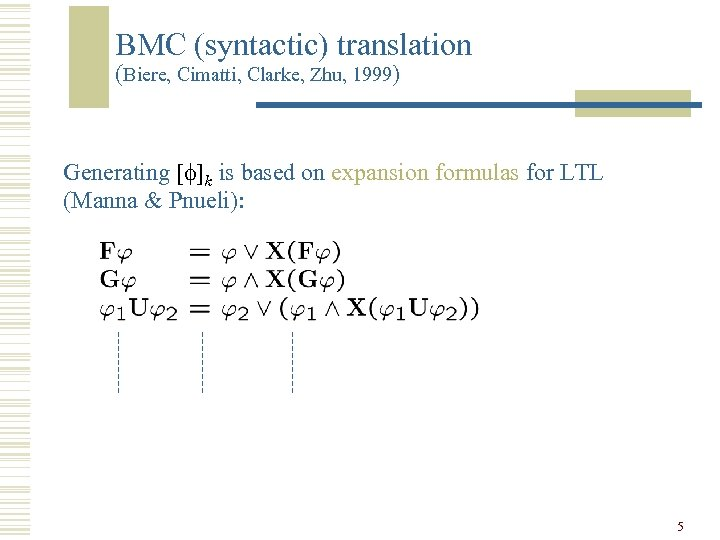BMC (syntactic) translation (Biere, Cimatti, Clarke, Zhu, 1999) Generating [ ]k is based on expansion formulas for LTL (Manna & Pnueli): 5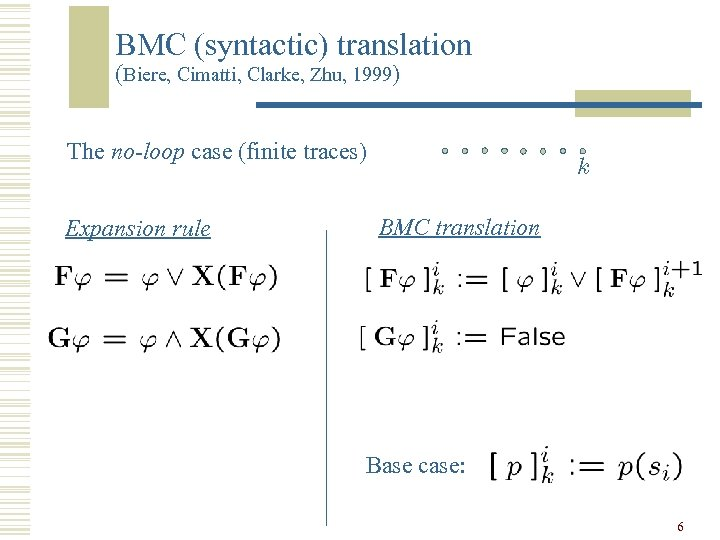BMC (syntactic) translation (Biere, Cimatti, Clarke, Zhu, 1999) The no-loop case (finite traces) Expansion rule k BMC translation Base case: 6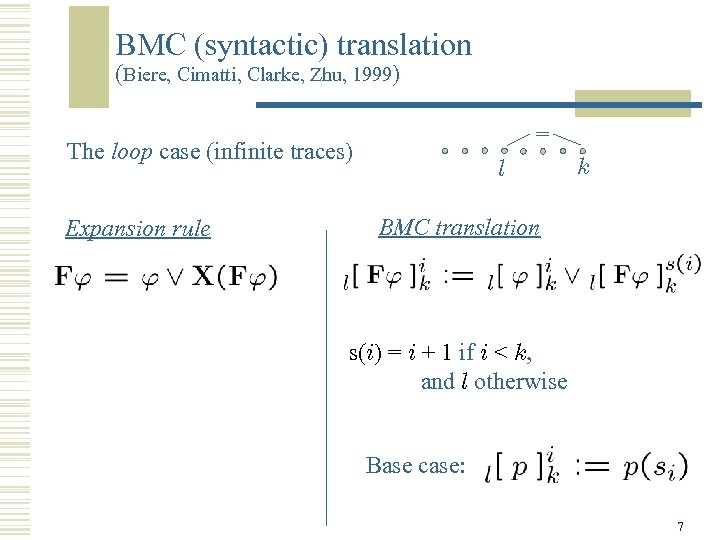BMC (syntactic) translation (Biere, Cimatti, Clarke, Zhu, 1999) = The loop case (infinite traces) Expansion rule l k BMC translation s(i) = i + 1 if i < k, and l otherwise Base case: 7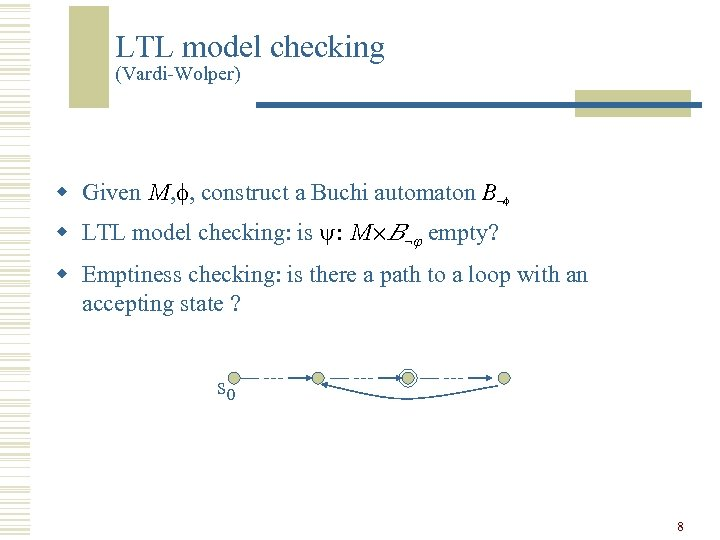LTL model checking (Vardi-Wolper) w Given M, , construct a Buchi automaton B w LTL model checking: is : M£B empty? w Emptiness checking: is there a path to a loop with an accepting state ? s 0 8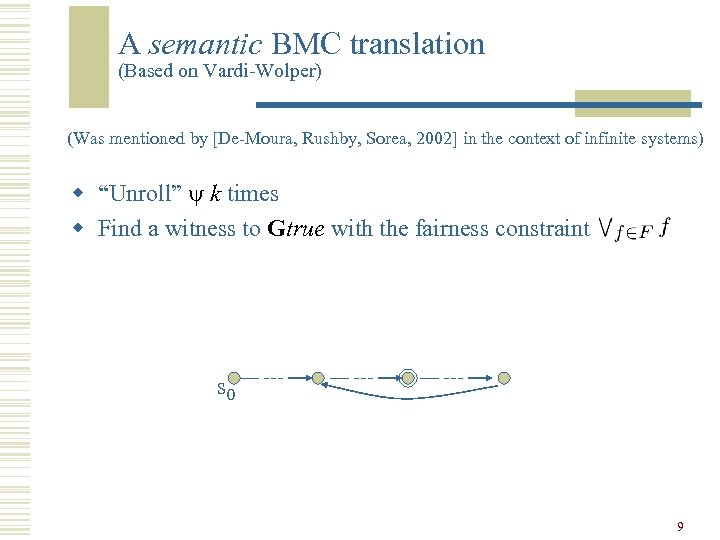A semantic BMC translation (Based on Vardi-Wolper) (Was mentioned by [De-Moura, Rushby, Sorea, 2002] in the context of infinite systems) w “Unroll” k times w Find a witness to Gtrue with the fairness constraint s 0 9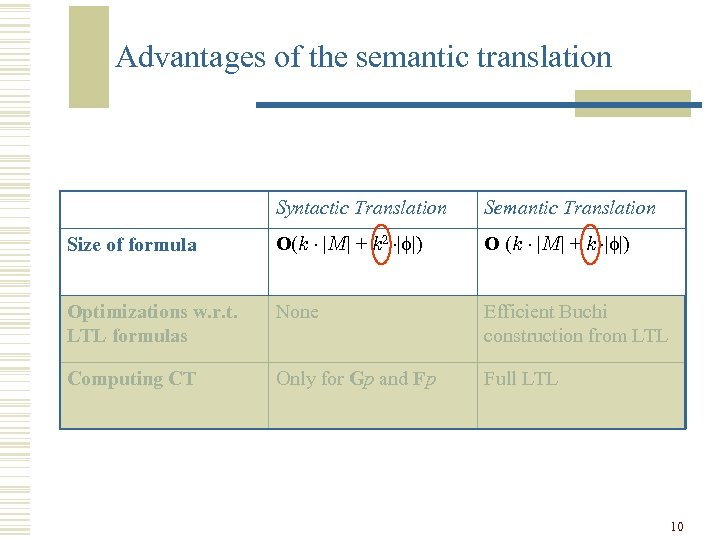Advantages of the semantic translation Syntactic Translation Semantic Translation Size of formula O(k ¢ |M| + k 2 ¢| |) O (k ¢ |M| + k ¢| |) Optimizations w. r. t. LTL formulas None Efficient Buchi construction from LTL Computing CT Only for Gp and Fp Full LTL 10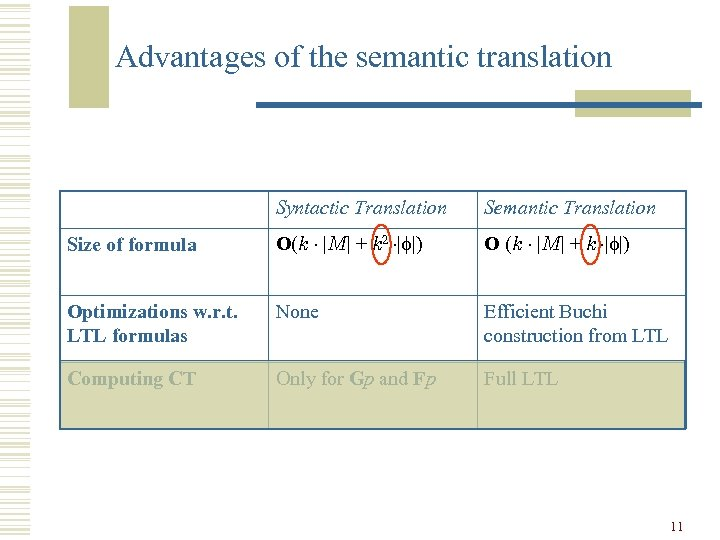Advantages of the semantic translation Syntactic Translation Semantic Translation Size of formula O(k ¢ |M| + k 2 ¢| |) O (k ¢ |M| + k ¢| |) Optimizations w. r. t. LTL formulas None Efficient Buchi construction from LTL Computing CT Only for Gp and Fp Full LTL 11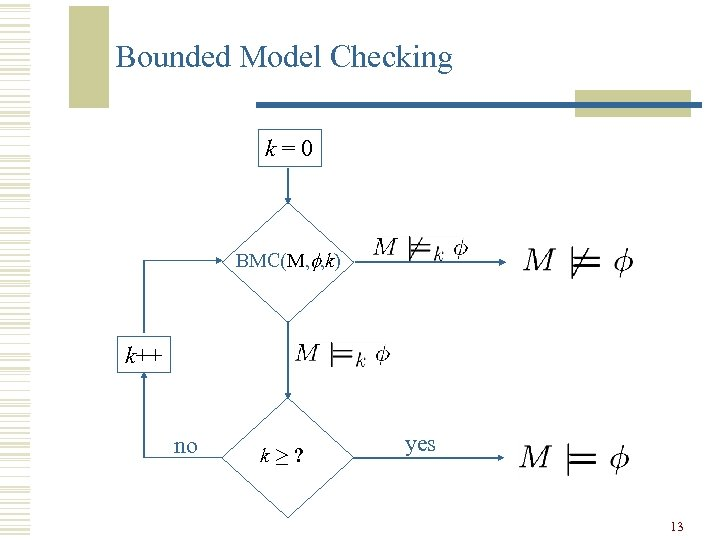Bounded Model Checking k=0 BMC(M, f, k) k++ no k¸? yes 13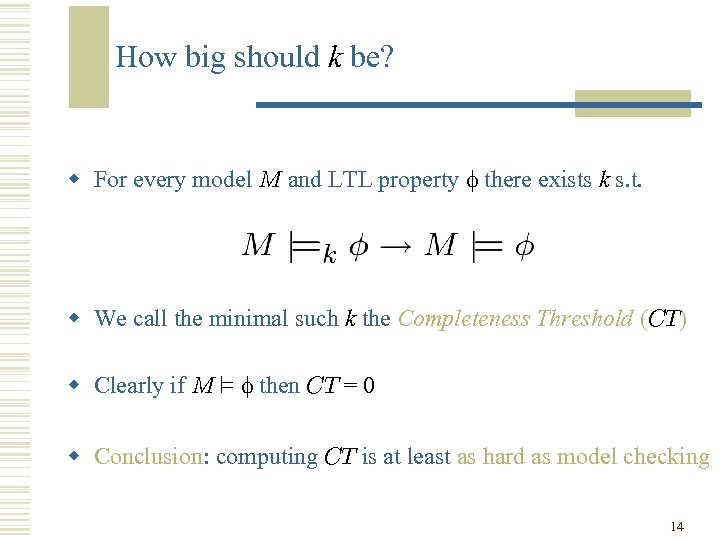How big should k be? w For every model M and LTL property there exists k s. t. w We call the minimal such k the Completeness Threshold (CT) w Clearly if M ² then CT = 0 w Conclusion: computing CT is at least as hard as model checking 14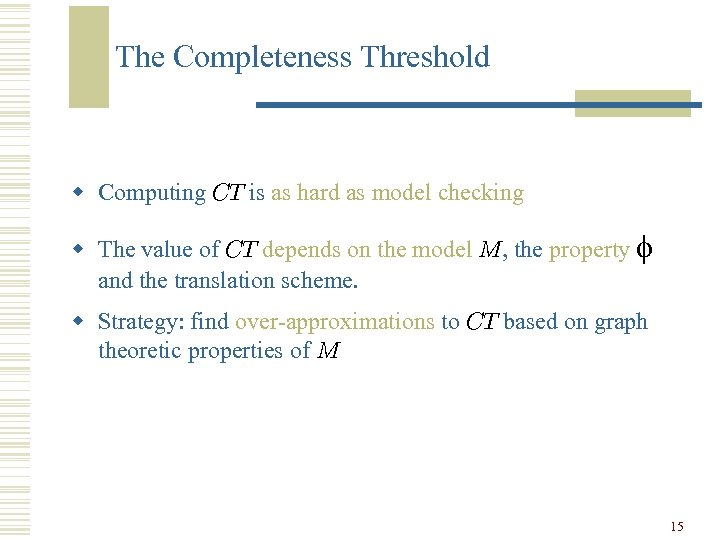The Completeness Threshold w Computing CT is as hard as model checking w The value of CT depends on the model M, the property and the translation scheme. w Strategy: find over-approximations to CT based on graph theoretic properties of M 15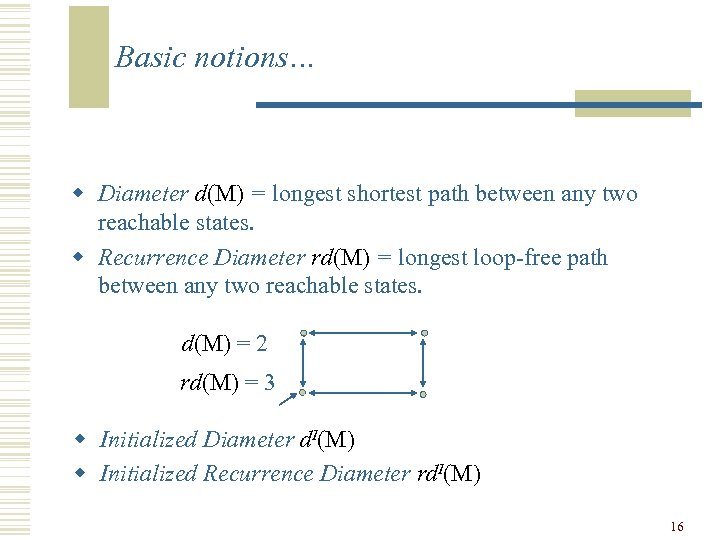Basic notions… w Diameter d(M) = longest shortest path between any two reachable states. w Recurrence Diameter rd(M) = longest loop-free path between any two reachable states. d(M) = 2 rd(M) = 3 w Initialized Diameter d. I(M) w Initialized Recurrence Diameter rd. I(M) 16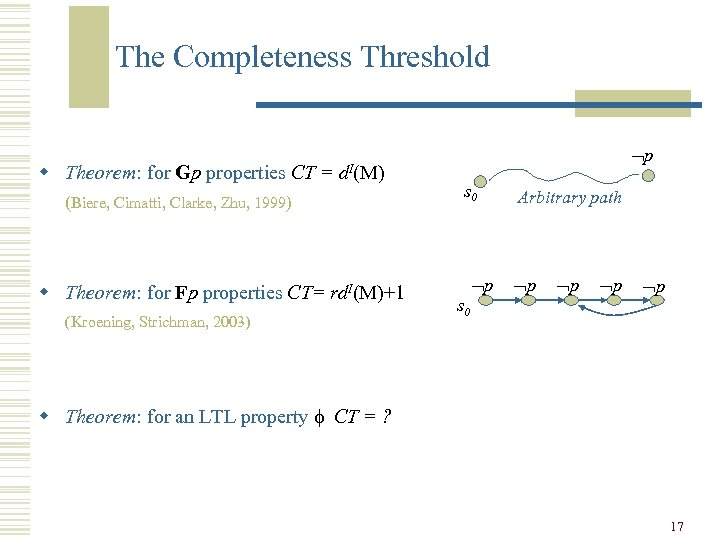The Completeness Threshold w Theorem: for Gp properties CT = d. I(M) (Biere, Cimatti, Clarke, Zhu, 1999) w Theorem: for Fp properties CT= rd. I(M)+1 (Kroening, Strichman, 2003) p s 0 Arbitrary path p p w Theorem: for an LTL property CT = ? 17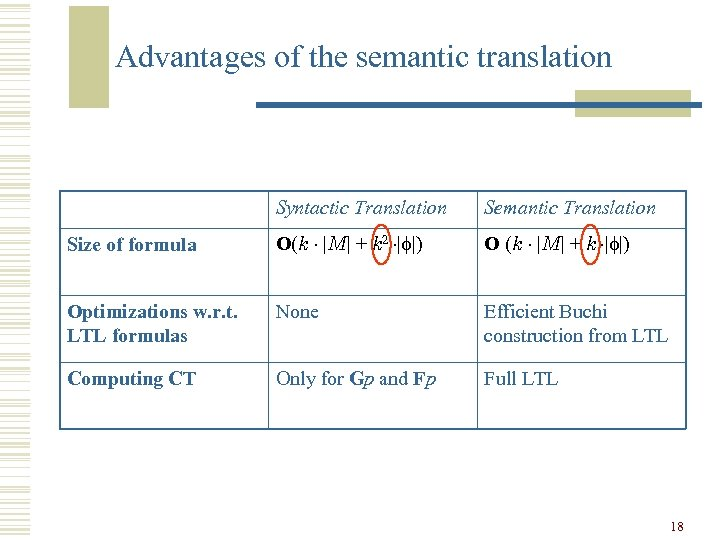Advantages of the semantic translation Syntactic Translation Semantic Translation Size of formula O(k ¢ |M| + k 2 ¢| |) O (k ¢ |M| + k ¢| |) Optimizations w. r. t. LTL formulas None Efficient Buchi construction from LTL Computing CT Only for Gp and Fp Full LTL 18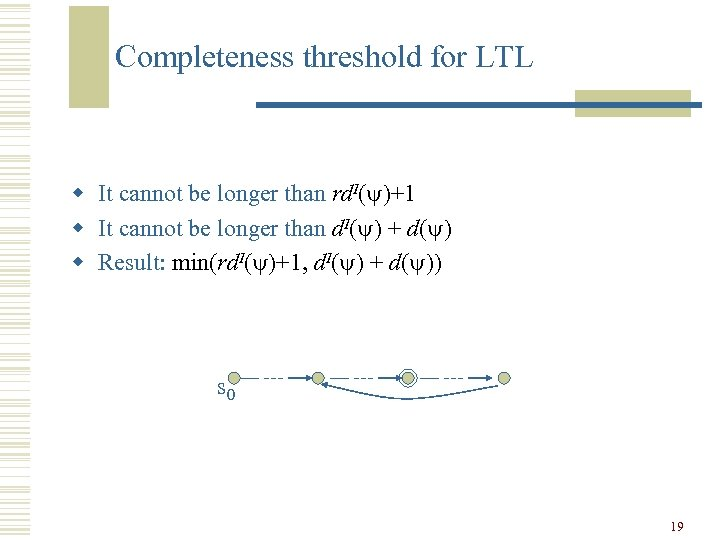Completeness threshold for LTL w It cannot be longer than rd. I( )+1 w It cannot be longer than d. I( ) + d( ) w Result: min(rd. I( )+1, d. I( ) + d( )) s 0 19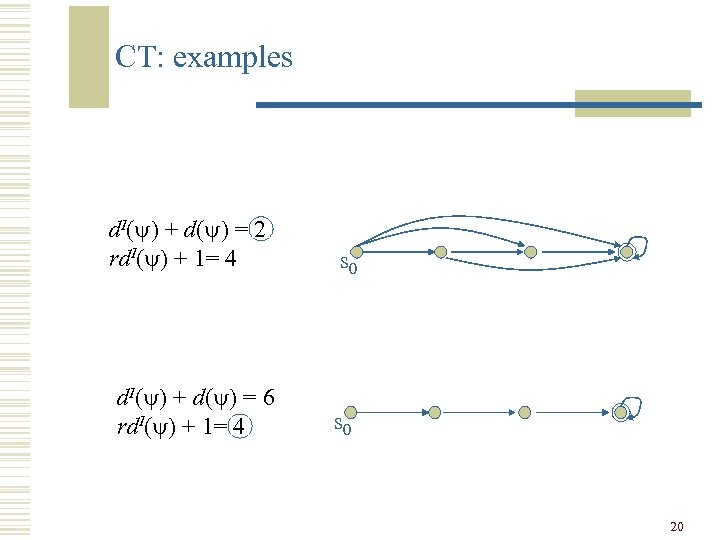CT: examples d. I( ) + d( ) = 2 rd. I( ) + 1= 4 d. I( ) + d( ) = 6 rd. I( ) + 1= 4 s 0 20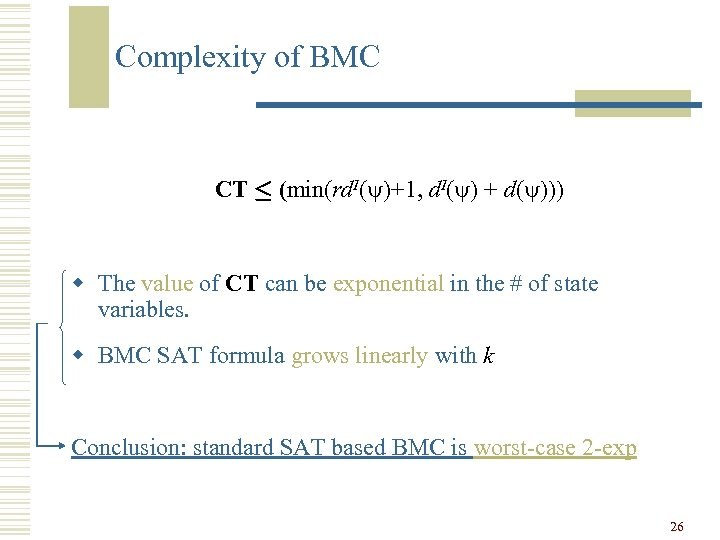Complexity of BMC CT · (min(rd. I( )+1, d. I( ) + d( ))) w The value of CT can be exponential in the # of state variables. w BMC SAT formula grows linearly with k Conclusion: standard SAT based BMC is worst-case 2 -exp 26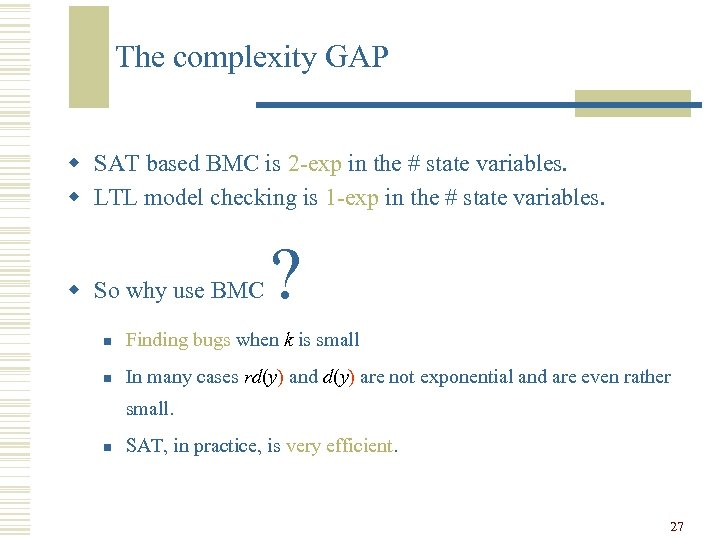The complexity GAP w SAT based BMC is 2 -exp in the # state variables. w LTL model checking is 1 -exp in the # state variables. w So why use BMC ? n Finding bugs when k is small n In many cases rd(y) and d(y) are not exponential and are even rather small. n SAT, in practice, is very efficient. 27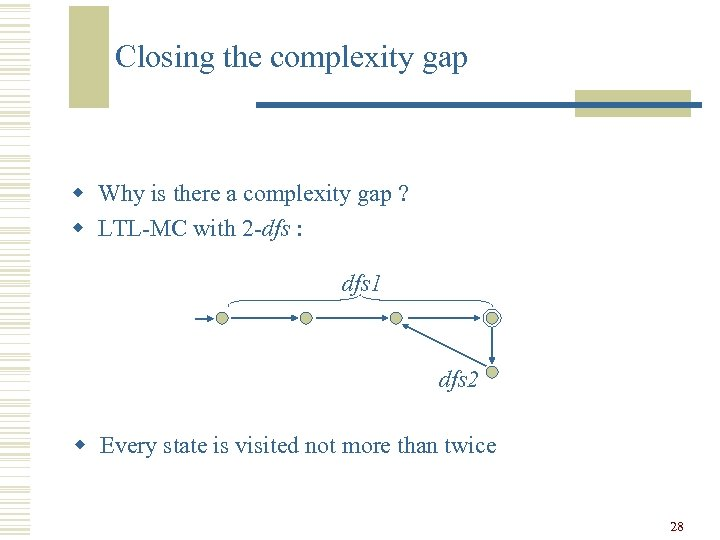Closing the complexity gap w Why is there a complexity gap ? w LTL-MC with 2 -dfs : dfs 1 dfs 2 w Every state is visited not more than twice 28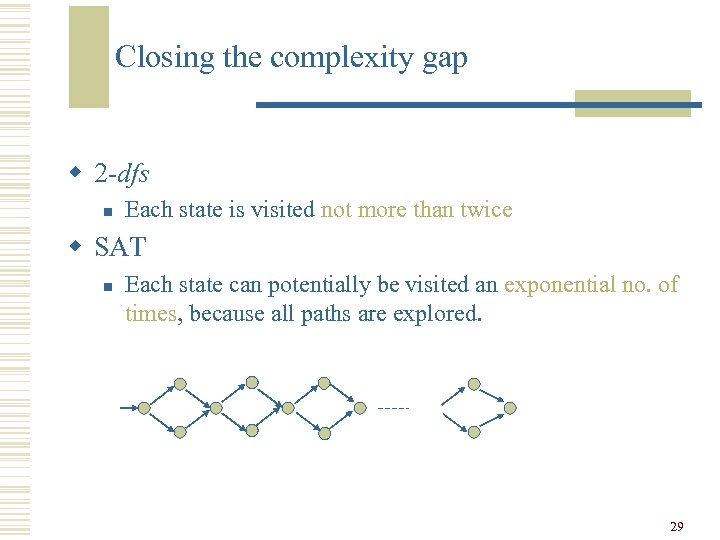Closing the complexity gap w 2 -dfs n Each state is visited not more than twice w SAT n Each state can potentially be visited an exponential no. of times, because all paths are explored. 29Closing the complexity gap (for Gp) w Force a static order, following a forward traversal w Each time a state i is fully evaluated (assigned): n n n Prevent the search from revisiting it through deeper paths (by adding conflict clauses) When backtracking from state i, prevent the search from revisiting it in step i If : pi holds stop and return “Counterexample found” 30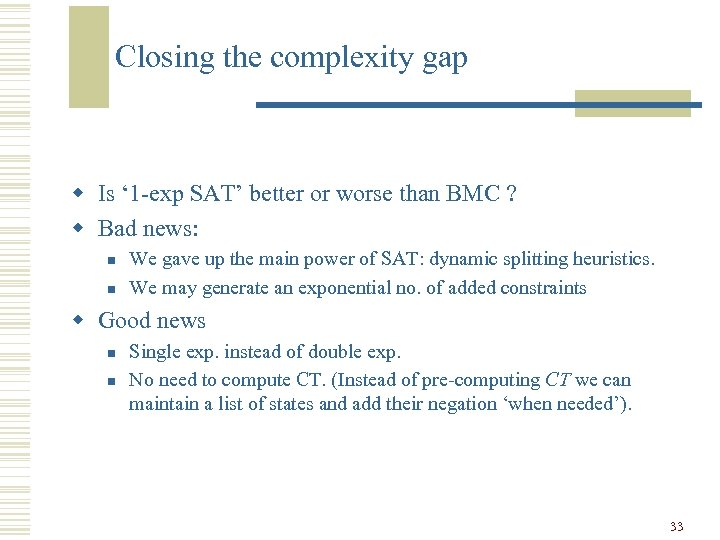Closing the complexity gap w Is ‘ 1 -exp SAT’ better or worse than BMC ? w Bad news: n n We gave up the main power of SAT: dynamic splitting heuristics. We may generate an exponential no. of added constraints w Good news n n Single exp. instead of double exp. No need to compute CT. (Instead of pre-computing CT we can maintain a list of states and add their negation ‘when needed’). 33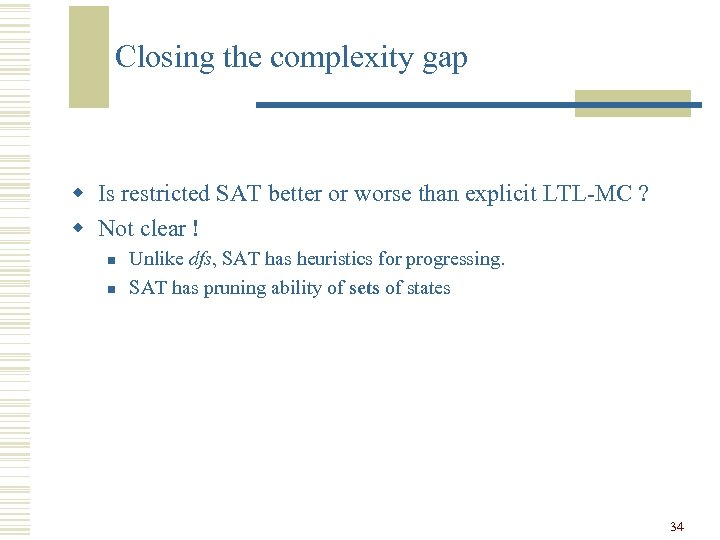Closing the complexity gap w Is restricted SAT better or worse than explicit LTL-MC ? w Not clear ! n n Unlike dfs, SAT has heuristics for progressing. SAT has pruning ability of sets of states 34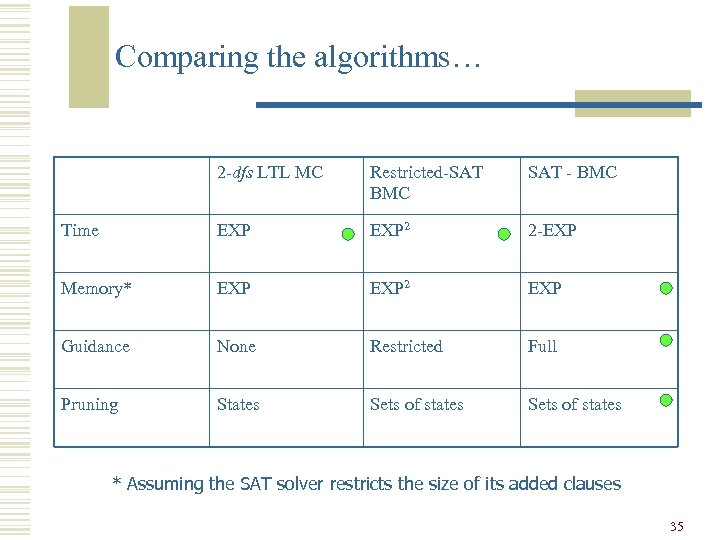Comparing the algorithms… 2 -dfs LTL MC Restricted-SAT BMC SAT - BMC Time EXP 2 2 -EXP Memory* EXP 2 EXP Guidance None Restricted Full Pruning States Sets of states * Assuming the SAT solver restricts the size of its added clauses 35